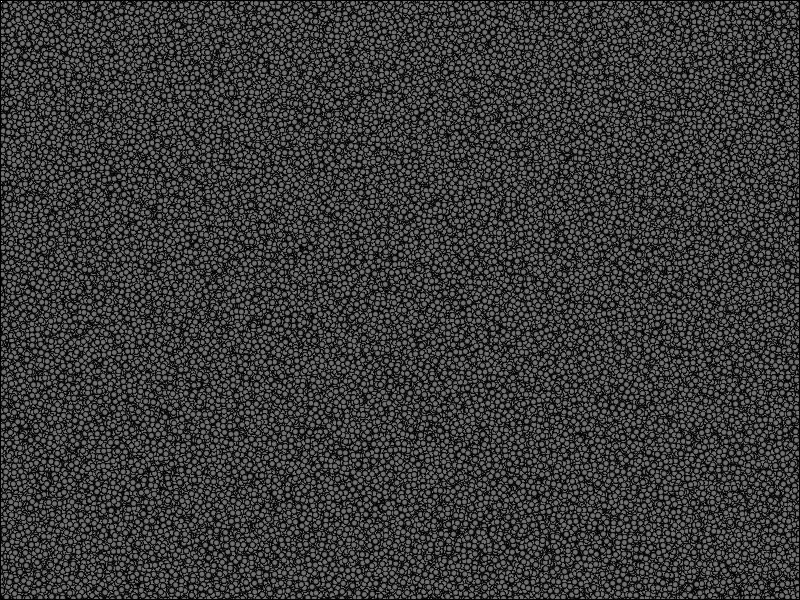# Vispy Lasso#

Demonstrate the use of lasso selection.

The lasso selection is done on a 2D scatter but could be extended further by user.```import sys
import warnings
import numpy as np
from vispy import app, scene
from vispy.geometry import curves
from vispy.scene import visuals

try:
from matplotlib import path
except ImportError:
warnings.warn("Lasso example requires matplotlib for more accurate selection. Falling back to numpy based selection.")
path = None

LASSO_COLOR               = (1, .1, .1)
FILTERED_COLOR            = (1, 1, 1, 0.3)
SELECTED_COLOR            = (0.3, 0, 1, 1.0)
MIN_MOVE_UPDATE_THRESHOLD = 5
NUMBER_POINT              = 2000000
SCATTER_SIZE              = 5

canvas = scene.SceneCanvas(keys='interactive', show=True)

num_segments=10, parent=view.scene)

lasso = scene.visuals.Line(pos=np.array([[0, 0], [0, 0]]), color = LASSO_COLOR, parent=view.scene, width = PEN_RADIUS , antialias=True)
px, py = 0, 0

# generate data
pos = 360 * np.random.normal(size=(NUMBER_POINT, 2), scale=1)

# one could stop here for the data generation, the rest is just to make the
# data look more interesting. Copied over from magnify.py
centers = np.random.normal(size=(NUMBER_POINT, 2), scale = 1) * 960
indexes = np.random.normal(size=NUMBER_POINT, loc=centers.shape / 2,
scale=centers.shape / 3)
indexes = np.clip(indexes, 0, centers.shape - 1).astype(int)
pos += centers[indexes]

# create scatter object and fill in the data
scatter = visuals.Markers()
point_color = np.full((NUMBER_POINT, 4), FILTERED_COLOR)
scatter.set_data(pos, edge_width=0, face_color=point_color, size=SCATTER_SIZE)

def points_in_polygon(polygon, pts):
"""Get boolean mask of points in a polygon reusing matplotlib implementation.

The fallback code is based from StackOverflow answer by ``Ta946`` in this question:
https://stackoverflow.com/questions/36399381/whats-the-fastest-way-of-checking-if-a-point-is-inside-a-polygon-in-python

This is a proof of concept and depending on your use case, willingness
on the above question would serve you better (ex. shapely, etc).
"""
# Filter vertices out of the polygon's bounding box, this serve as an early optimization whenever number of vertices
# to filter out is huge.
x1, x2, y1, y2 = min(polygon[:, 0]), max(polygon[:, 0]), min(polygon[:, 1]), max(polygon[:, 1])
selection_mask = (x1 < pts[:, 0]) & (pts[:, 0] < x2) & (y1 < pts[:, 1]) & (pts[:, 1] < y2)

# Select vertices inside the polygon.
if path is not None:
polygon = path.Path(polygon[:, :2], closed = True)
else:
contour2 = np.vstack((polygon[1:], polygon[:1]))
test_diff = contour2-polygon
m1 = (polygon[:,1] > pts_in_bbox[:,None,1]) != (contour2[:,1] > pts_in_bbox[:,None,1])
slope = ((pts_in_bbox[:,None,0]-polygon[:,0])*test_diff[:,1])-(test_diff[:,0]*(pts_in_bbox[:,None,1]-polygon[:,1]))
m2 = slope == 0
m3 = (slope < 0) != (contour2[:,1] < polygon[:,1])
m4 = m1 & m3
count = np.count_nonzero(m4, axis=-1)

# Return the full selection mask based on bounding box & polygon selection.

def select(polygon_vertices, points):
# Set default mask to filter everything since user selection
# is not yet calculated.

if polygon_vertices is not None:
# Optimization: It's faster to convert lasso selection straight to visual coordinates since there's generally less vertices
# this would speed up the processing depending on the scene.
polygon_vertices = scatter.get_transform('canvas', 'visual').map(polygon_vertices)

@canvas.connect
def on_mouse_press(event):

if event.button == 1:
# Reset lasso state.
lasso.set_data(pos=np.empty((1, 2)))

# Reset selected vertices to the filtered color, this would earn some time in case
# scene contains a lot of vertices.

scatter.set_data(pos, edge_width=0, face_color=point_color, size=SCATTER_SIZE)

@canvas.connect
def on_mouse_move(event):
global pointer, px, py

pp = event.pos

# Optimization: to avoid too much recalculation/update we can update scene only if the mouse
# moved a certain amount of pixel.
if (abs(px - pp) > MIN_MOVE_UPDATE_THRESHOLD or abs(py - pp) > MIN_MOVE_UPDATE_THRESHOLD):
pointer.center = pp
if event.button == 1:
polygon_vertices = event.trail()
lasso.set_data(pos = np.insert(polygon_vertices, len(polygon_vertices), polygon_vertices, axis=0))
px, py = pp

@canvas.connect
def on_mouse_release(event):

if event.button == 1:

# Set selected points with selection color